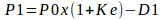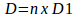How to Calculate Dividend Decision

What Is Dividend Decision?

The dividend is the amount of money paid by the company to its investors. The dividend payments are calculated as dividend per share multiply by a number of outstanding shares.Assignment:

X Ltd., had 25,000 equity shares of Rs. 100 each outstanding on 1st April, the
shares are issued at par in the market, the company removed restraint in the
dividend policy, the company ready to pay dividend of Rs. 15 per share for the
current calendar year. The capitalization rate is 15%. Using MM approach assuming
that no taxes, calculate the price of the shares at the end of the year:
(a) When dividend is not declared.
(b) When dividend is declared.
(c) Find out the number of new shares that the company issues to meet its
investment needs of Rs 15,00,000 assuming that net income of Rs. 7,50,000
and assuming that the dividend is paid.

Using the MM approach, the price of the share (P1) at the end of the period will be defined as a following:Where:
P0 = Prevailing market price of a share K e = Cost of equity capital

D1 = Dividend at the end of the first period

1. Calculation price of a share at the end of the period when dividend is not declared

If the dividends are not declared, the company hasn’t any obligation to its investors, and the dividends will be equal zero. P1 = Rs.100 x (1+0.15)-0 = Rs.115

Price of a share at the end of the period when dividend declaration is avoided is Rs. 115.

1. Calculation price of a share at the end of the period when dividend is declared

If the dividends are declared of Rs. 15, the price of a share at the end of the period will be calculated as a following: P1 = Rs.100 x (1+0.15)-15 = Rs.100

Price of a share at the end of the period when dividend declaration is present is Rs. 100.

1. Finding out the number of new share for new investment of Rs.15,00,000

For finding the required number of share for investment need, the formula will be used:Where:

M = Number of new shares to be issued

P1 = Price at which new issue is to be made

I = Amount of investment required

X = Net profit earned for period

nD1 = Dividend paid for the period

Step 1

Using the data of requirement #b, the price of share at which new shares will be issued equals R s.100

Step 2

The annual dividend (D) will be calculated using the following formula:Where:

n = Number of outstanding shares at April 1

D1 = Dividend per share

D =25,000 x Rs.15 =Rs.3,75,000

Step 3

If the required investment equals Rs.15,00,000 and net income for the period is Rs.7,50,000, the number of new share for new investment (M) will be computed:M = (15,00,000-(7,50,000-3,75,000))/100 = 11,25,000/100 = 11,250

The number of new share for the new investment of Rs.15,00,000 equal 11,250 shares.